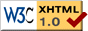# Draft Proposal for UTF-E-32 Specification

Authors: Tom Bishop (tbishop@wenlin.com) and Richard Cook (rscook@wenlin.com).

Updated October, 2009

Please Note: This is not a finalized specification. It is still at the "draft proposal" stage and may change.

## 1. Introduction

The name of this UTF (UCS Transformation Format) is "UTF-E-32". It extends UTF-32 to support code points up to U+7FFFFFFFFFFFFFFF.

UTF-E-32 is one of the encodings defined as part of UCS-E, which also includes similar extensions for UTF-8 and UTF-16. For general information about UCS-E, please see the UCS-E Specification.

## 2. Properties

UTF-E-32 preserves and extends useful properties of UTF-32 and UCS-4. For code points less than or equal to U+10FFFF, it is identical to UTF-32. For code points less than U+80000000, it is identical to the original UCS-4 encoding.

UTF-E-32 employs thirty-two-bit code units. Each code is one, two, or three units in length. For codes that consist of two or three code units, the leading (initial) code unit always matches the pattern Fxxxxxxx (hexadecimal), and all trailing (non-initial) code units match the pattern Exxxxxxx (hexadecimal). (Hexadecimal notation is used for code points and code units, except where binary or decimal are explicitly specified.) The distinction between leading and trailing code units has the consequence that there is no risk of a "false match" when searching.

A simple binary comparison of UTF-E-32 codes yields the same sort-order as a numerical comparison of code points.

UTF-E-32 specifies the code length in the first code unit.

## 3. Details

(Note: some readers may prefer to skip down to view the examples first, then return here to read the details.)

### 3.1 Terms and Abbreviations

• Code point: Any nonnegative integer used for character encoding.
• USV: A UCS-E scalar value, that is, any code point in the range U+0000..7FFFFFFFFFFFFFFF except U+D800..DFFF.
• Udigit: A USV hexadecimal digit used in the conventional "U+" notation.
• NUD: Number of udigits in a particular USV, not counting leading zeros. For example, U+123456789 has NUD = 9, and U+0050 has NUD = 2.
• Nybble: Four bits; one hexadecimal digit.
• Unit: A thirty-two-bit (eight-nybble) code unit that forms part or all of a UTF-E-32 code.
• Code: A whole sequence of UTF-E-32 units corresponding to a single code point.

### 3.2 Code points less than or equal to U+DFFFFFFF

A code is a single unit which simply contains the USV.

### 3.3 Code points in the range U+E0000000..U+DFFFFFFFFFFFFF

A code consists of two units. The USV is padded on the left with zeros as needed to make fourteen nybbles. The leading unit has the form Fxxxxxxx and stores the first seven nybbles; it is in the range F000000E..FDFFFFFF. The trailing unit has the form Exxxxxxx and stores the last seven nybbles.

### 3.4 Code points in the range U+E0000000000000..U+7FFFFFFFFFFFFFFF

A code consists of three units. The leading unit has the form FF0000xx, and is in the range FF000000..FF00007F. Both trailing units have the form Exxxxxxx. The USV is padded on the left with zeros as needed to make sixteen nybbles, of which two are stored in the first unit, seven in the second unit, and seven in the third unit.

### 3.5 Analysis

1 unitU+0000..U+DFFFFFFF00000000..DFFFFFFF(none)
2 unitsU+E0000000..U+DFFFFFFFFFFFFFF000000E..FDFFFFFFE0000000..EFFFFFFF
3 unitsU+E0000000000000..U+7FFFFFFFFFFFFFFFFF000000..FF00007F

## 4. Examples

``` U+0041 = 00000041 (one unit; the code for the letter 'A')

U+10FFFF = 0010FFFF (one unit; the last UTF-32 code)

U+110000 = 00110000 (one unit)

U+7FFFFFFF = 7FFFFFFF (one unit; the last original UCS-4 code)

U+80000000 = 80000000 (one unit)

U+DFFFFFFF = DFFFFFFF (the last one-unit code)

U+E0000000 = F000000E E0000000 (the first two-unit code)

U+123456789ABCD = F0123456 E789ABCD (two units)

U+DFFFFFFFFFFFFF = FDFFFFFF EFFFFFFF (the last two-unit code; NUD = fourteen)

U+E0000000000000 = FF000000 EF000000 E0000000
(the first three-unit code; NUD = fourteen)

U+123456789ABCDEF0 = FF000012 E3456789 EABCDEF0 (NUD = sixteen)

U+7FFFFFFFFFFFFFFF = FF00007F EFFFFFFF EFFFFFFF (the last code point in UCS-E)
```

To the UCS-E Specification